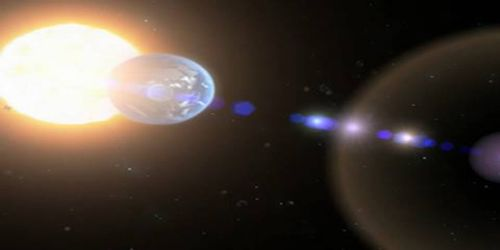Physics

# Theory of Relativity in Journey to SpaceTheory of Relativity in Journey to Space

Time dilation and length contraction phenomena happen in the journey to space. Due to travel to a long distance, even to make the journey to our nearest planet it takes a long time. The Alpha Centauri is the closed star to us which is 4.3 light year distance away. That means, light from this star if measured from a stationary observer on earth, takes 4.3 years. Suppose a rocket with a speed of 0.95c travels to Alpha Centauri. Here two things are involved. One is to go from the earth and the other one is to reach to Alpha Centauri. Just before going earth is outside the space shuttle and just after reaching Alpha Centauri is outside the space shuttle. So to the astronaut, the two events occur at the same place, i.e., outside the space shuttle.

To an observer on earth, the two events occur at two different places. So, time measured by a person, time dilation.

Δt = 4.3 / 0.95  year = 4.5 years

According to the time dilation theory, an actual time interval in watches of the astronaut will be,

Δt0 = Δt √[1 – (v2 / c2)] = 4.5 x √[1 – (0.95c/c)2]

= 14 years

So, when the astronauts will arrive at the Alpha Centauri, then their ages will increase by 1.4 years, but not 4.5 years as measured by the observer on earth.

Again, a rod is inside a rocket. When the rocket is in motion at a speed nearly to the speed of light, then if the length of the rod is measured inside the rocket, it will be seen that the length in motion is less than the length at rest. That means the length of the rod on earth at rest will be larger than the length in motion. If the length of a body in motion with respect to an observer is L and if the length of the same body at rest with respect to that observer is L0, then L will always be smaller than L0. Here L0 is called appropriate or proper length which is related by the following equation,

L = L0 √[1 – (v2 / c2)]

Here, v = velocity of the rocket and c = velocity of light.